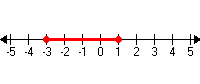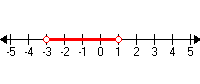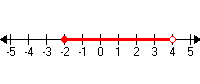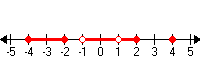# Interval Notation

Interval notation is a way of writing subsets of the real number line .

A closed interval is one that includes its endpoints: for example, the set $\left\{x\text{\hspace{0.17em}}|\text{\hspace{0.17em}}-3\le x\le 1\right\}$ .To write this interval in interval notation, we use closed brackets [ ]:

$\left[-3,1\right]$

An open interval is one that does not include its endpoints, for example, $\left\{x\text{\hspace{0.17em}}|\text{\hspace{0.17em}}-3 .To write this interval in interval notation, use parentheses :

$\left(-3,1\right)$

You can also have intervals which are half-open and half-closed:$\left[-2,4\right)$

You can also use interval notation together with the set union operator to write subsets of the number line made up of more than one interval:$\left[-4,-2\right]\cup \left(-1,1\right)\cup \left(1,2\right]\cup \left\{4\right\}$